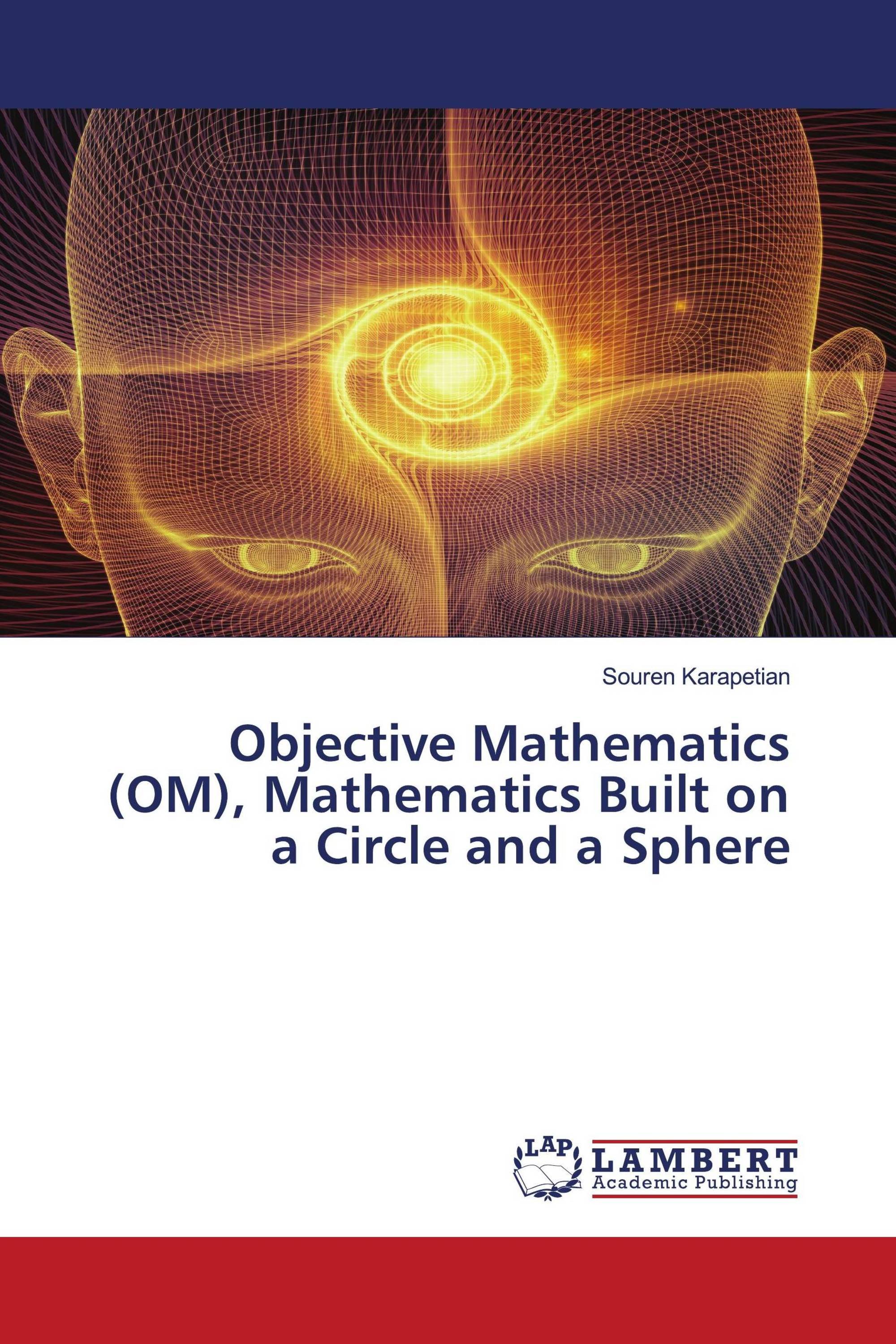﻿ Objective Mathematics (OM), Mathematics Built on a Circle and a Sphere / 978-620-3-86066-5 / 9786203860665 / 6203860662# Objective Mathematics (OM), Mathematics Built on a Circle and a Sphere

LAP Lambert Academic Publishing ( 2021-05-10 )

€ 26,90

The of this work asked himself: why is mathematics built on a mythical infinite straight line and plane, and what happens if it is built on a circle and a sphere - the most perfect objects of nature? The called the resulting theory Objective Mathematics (OM), bearing in mind that this theory operates on natural objects that exist in nature (a circle and a sphere) and does not use the axiomatic approach, in particular the infinite parallel straight lines and planes present in traditional mathematics (TM). The constructs and proofs in this article are first made on a circle (one-dimensional OM), and then the resulting law is generalized to a sphere (two-dimensional OM) and 3-sphere (three-dimensional OM).The results obtained 4 empirical laws and 21 laws.The paper gives definitions of such concepts as:• A harmonic four (quartet) on a circle,• A logical three-dimensional count,• Preliminary mathematics (Premathematics) in OM,• Non-Euclidean geometry in OM,• One-dimensional, two-dimensional, three-dimensional OM, etc.As a result of the analysis, the concludes that the universe is a three-dimensional sphere, where a ray of light is a large circle of this sphere.

### Book Details:

ISBN-13:

978-620-3-86066-5

ISBN-10:

6203860662

EAN:

9786203860665

Book language:

English

By () :

Souren Karapetian

Number of pages:

56

Published on:

2021-05-10

Category:

Mathematics